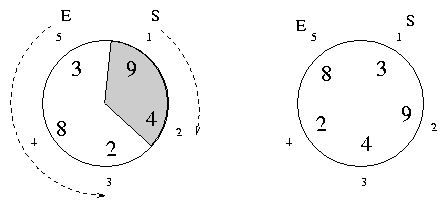## Saturday, August 28, 2010

### poj_1247_Magnificent_Meatballs.cp

poj1247, nit1174,

## Problem:

Magnificent Meatballs
 Time Limit: 1000MS Memory Limit: 10000K Total Submissions: 4574 Accepted: 3080
Description
Sam and Ella run a catering service. They like to put on a show when serving meatballs to guests seated at round tables. They march out of the kitchen with pots of meatballs and start serving adjacent guests. Ella goes counterclockwise and Sam goes clockwise, until they both plop down their last meatball, at the same time, again at adjacent guests. This impressive routine can only be accomplished if they can divide the table into two sections, each having the same number of meatballs. You are to write a program to assist them.

At these catering events, each table seats 2 <= N <= 30 guests. Each guest orders at least one and at most nine meatballs. Each place at the table is numbered from 1 to N, with the host at position 1 and the host's spouse at position N. Sam always serves the host first then proceeds to serve guests in increasing order. Ella serves the spouse first, then serves guests in decreasing order. The figures illustrate the first two example input cases.Input
Input consists of one or more test cases. Each test case contains the number of guests N followed by meatballs ordered by each guest, from guest 1 to guest N. The end of the input is a line with a single zero.
Output
For each table, output a single line with the ending positions for Sam and Ella, or the sentence indicating an equal partitioning isn't possible. Use the exact formatting shown below.
Sample Input
```5 9 4 2 8 3
5 3 9 4 2 8
6 1 2 1 2 1 2
6 1 2 1 2 1 1
0```
Sample Output
```Sam stops at position 2 and Ella stops at position 3.
No equal partitioning.
No equal partitioning.
Sam stops at position 3 and Ella stops at position 4.```
Source
Mid-Central USA 2002

## Source Code:

//Sat Jul  3 22:32:26 CDT 2010
#include <vector>
#include <list>
#include <map>
#include <set>
#include <deque>
#include <queue>
#include <stack>
#include <bitset>
#include <algorithm>
#include <functional>
#include <numeric>
#include <utility>
#include <sstream>
#include <iostream>
#include <iomanip>
#include <cstdio>
#include <cmath>
#include <cstdlib>
#include <cctype>
#include <string>
#include <cstring>
#include <cstdio>
#include <cmath>
#include <cstdlib>
#include <ctime>

using namespace std;

int main(int argc, const char* argv[])
{
//  freopen("input.in", "r", stdin);
//  freopen("output.out", "w", stdout);
int N;
while (cin >> N)
{
if (N == 0)
break;
vector<int> v(N);
for (int i = 0; i < N; i++)
{
cin >> v[i];
}
int sum1 = 0;
int sum2 = 0;
for (int i = 1; i <= N; i++)
{
sum1 = 0;
sum2 = 0;
for (int p = 0; p < i; p++)
sum1 += v[p];
for (int p = i; p < N; p++)
sum2 += v[p];
if (sum2 == sum1)
{
cout << "Sam stops at position " << i
<< " and Ella stops at position " << (i + 1) << "."
<< endl;
break;
}
}
if (sum1 != sum2)
cout << "No equal partitioning." << endl;
}
//  fclose(stdin);
//  fclose(stdout);
return 0;
}